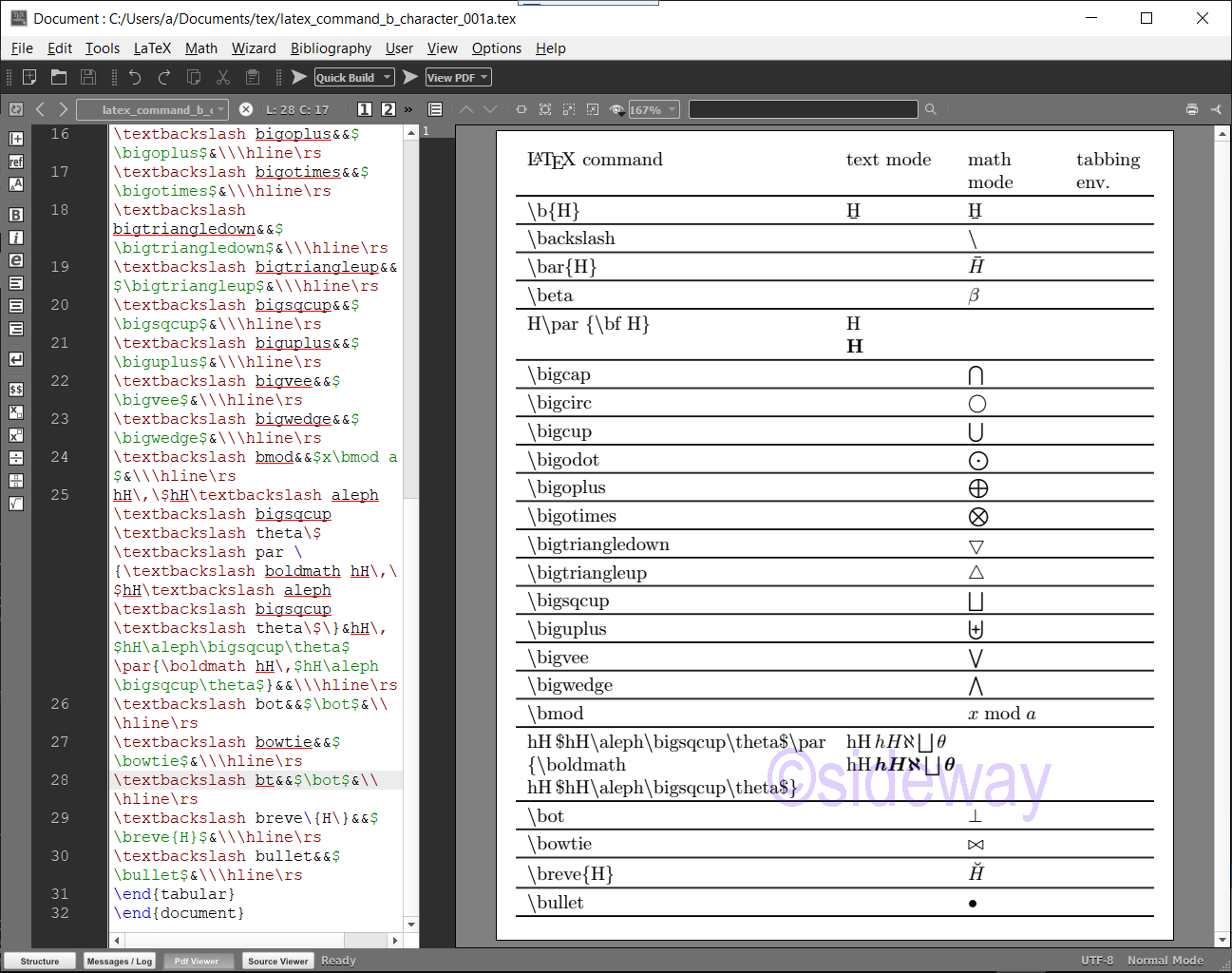output.to from Sideway
Draft for Information Only

# Content

LaTeX \b⋯ Commands for Printing Characters \b⋯ Commands \b⋯ Command Examples Source and Reference

# LaTeX \b⋯ Commands for Printing Characters

LaTeX uses backslash+b⋯ combination to print characters.

## \b⋯ Commands

The typical \b⋯ Commands for Printing Characters are LaTeX CommandsRemarksMode \bmakes a bar below accent. e.g. Ḇ, ḇ. \backslashprints a backslash, \.math mode \barmakes a macron over a letter. e.g. Ā, ā.math mode \betaprints a Greek small letter Beta, β.math mode \bfprints bold face type. \bigcapprints a N-ary intersection, ⋂.math mode \bigcircprints a heavy circle, ⭘.math mode \bigcupprints a N-ary union, ⋃.math mode \bigodotprints a N-ary circled dot operator, ⨀.math mode \bigoplusprints a N-ary circled plus operator, ⨁.math mode \bigotimesprints a N-ary circled times operator, ⨂.math mode \bigtriangledownprints a white down pointing triangle, ▽.math mode \bigtriangleupprints a white up pointing triangle, △.math mode \bigsqcupprints a N-ary square union operator, ⨆.math mode \biguplusprints a N-ary union operator with plus, ⨄.math mode \bigveeprints a N-ary logical or, ⋁.math mode \bigwedgeprints a N-ary logical and, ⋀.math mode \bmodprints a binary modulo expression, x mod a, with a short gap between x and mod.math mode \boldmathprints bold face type of math italics and math symbols only. Shoild be used outside of math mode. \botprints a up tack or falsum, ⊥.math mode \bowtieprints a bowtie, ⋈.math mode \brevemakes a breve accent. e.g. Ă, ă.math mode \bulletprints a bullet, •.math mode

## \b⋯ Command Examples

Examples of LaTeX commands
###### Code
\documentclass[border=10pt,varwidth]{standalone}
\newcommand\rs{\hline\noalign{\vskip 0.2em}}
\newenvironment{tabtest} {\setlength{\topsep}{0mm}\setlength{\partopsep}{0mm}\begin{tabbing}\hspace*{9mm}\kill}{\end{tabbing} }
\begin{document}
\begin{tabular}{p{0.45\textwidth}p{.15\textwidth}p{.13\textwidth}p{.1\textwidth}}
\LaTeX\ command & text mode & math mode & tabbing env.\\\hline\rs
\textbackslash b\{H\}&\b{H}&$\b{H}$&\\\hline\rs
\textbackslash backslash&&$\backslash$&\\\hline\rs
\textbackslash bar\{H\}&&$\bar{H}$&\\\hline\rs
\textbackslash beta&&$\beta$&\\\hline\rs
H\textbackslash par \{\textbackslash bf H\}&H\par{\bf H}&&\\\hline\rs
\textbackslash bigcap&&$\bigcap$&\\\hline\rs
\textbackslash bigcirc&&$\bigcirc$&\\\hline\rs
\textbackslash bigcup&&$\bigcup$&\\\hline\rs
\textbackslash bigodot&&$\bigodot$&\\\hline\rs
\textbackslash bigoplus&&$\bigoplus$&\\\hline\rs
\textbackslash bigotimes&&$\bigotimes$&\\\hline\rs
\textbackslash bigtriangledown&&$\bigtriangledown$&\\\hline\rs
\textbackslash bigtriangleup&&$\bigtriangleup$&\\\hline\rs
\textbackslash bigsqcup&&$\bigsqcup$&\\\hline\rs
\textbackslash biguplus&&$\biguplus$&\\\hline\rs
\textbackslash bigvee&&$\bigvee$&\\\hline\rs
\textbackslash bigwedge&&$\bigwedge$&\\\hline\rs
\textbackslash bmod&&$x\bmod a$&\\\hline\rs
hH\,\$hH\textbackslash aleph\textbackslash bigsqcup\textbackslash theta\$\textbackslash par \{\textbackslash boldmath hH\,\$hH\textbackslash aleph\textbackslash bigsqcup\textbackslash theta\$\}&hH\,$hH\aleph\bigsqcup\theta$\par{\boldmath hH\,$hH\aleph\bigsqcup\theta$}&&\\\hline\rs
\textbackslash bot&&$\bot$&\\\hline\rs
\textbackslash bowtie&&$\bowtie$&\\\hline\rs
\textbackslash bt&&$\bot$&\\\hline\rs
\textbackslash breve\{H\}&&$\breve{H}$&\\\hline\rs
\textbackslash bullet&&$\bullet$&\\\hline\rs
\end{tabular}
\end{document}
###### Output## Source and Reference

©sideway

ID: 210200019 Last Updated: 2/19/2021 Revision: 0Home 5

Business

Management

HBR 3

Information

Recreation

Culture

Chinese 1097

English 337

Computer

Hardware 153

Software

Application 204

Latex 34

Manim 171

Numeric 19

Programming

Web 285

Unicode 504

HTML 65

CSS 59

ASP.NET 194

OS 409

Python 56

Knowledge

Mathematics

Algebra 30

Geometry 21

Calculus 67

Engineering

Mechanical

Rigid Bodies

Statics 92

Dynamics 37

Control

Natural Sciences

Electric 27

Copyright © 2000-2021 Sideway . All rights reserved Disclaimers last modified on 06 September 2019